Courses

# Legendre Special Function (Part - 8)- Mathematical Methods of Physics, UGC - NET Physics Physics Notes | EduRev

## Physics for IIT JAM, UGC - NET, CSIR NET

Created by: Akhilesh Thakur

## Physics : Legendre Special Function (Part - 8)- Mathematical Methods of Physics, UGC - NET Physics Physics Notes | EduRev

The document Legendre Special Function (Part - 8)- Mathematical Methods of Physics, UGC - NET Physics Physics Notes | EduRev is a part of the Physics Course Physics for IIT JAM, UGC - NET, CSIR NET.
All you need of Physics at this link: Physics

Qn(x ) Functions of the Second Kind

It will be noticed that we have used only pn for n even and qn for n odd (because they terminated for this choice of n). We may now deﬁne a second solution of Legendre’s equation (Fig. 12.17) by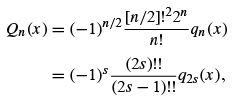for n even,n = 2s,           (12.215)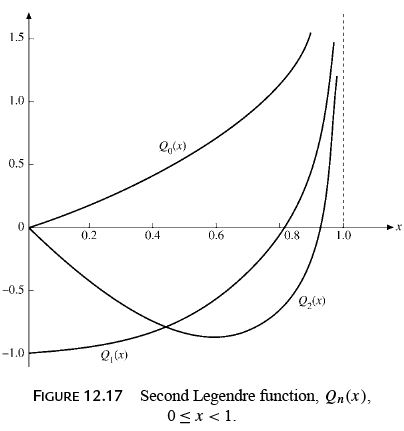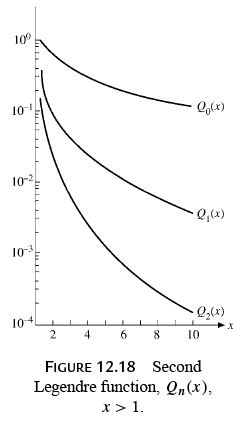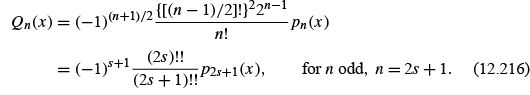This choice of normalizing factors forces Qn to satisfy the same recurrence relations as Pn . This may be veriﬁed by substituting Eqs. (12.215) and (12.216) into Eqs. (12.17) and (12.26). Inspection of the (series) recurrence relations (Eqs. (12.207) and (12.209)), that is, by the Cauchy ratio test, shows that Qn (x ) will converge for −1 <x < 1. If |x |≥ 1, these series forms of our second solution diverge. A solution in a series of negative powers of x can be developed for the region |x | > 1 (Fig. 12.18), but we proceed to a closed-form solution that can be used over the entire complex plane (apart from the singular points x =±1 and with care on cut lines).

Closed-Form Solutions

Frequently, a closed form of the second solution, Q(z), is desirable. This may be obtained by the method discussed in Section 9.6. We write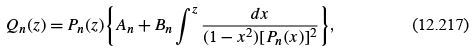in which the constant An replaces the evaluation of the integral at the arbitrary lower limit.
Both constants, An and Bn , may be determined for special cases.

For n = 0, Eq. (12.217) yields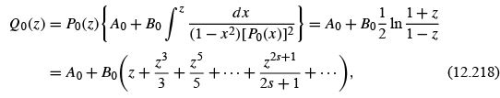the last expression following from a Maclaurin expansion of the logarithm. Comparing this with the series solution (Eq. (12.210)), we obtain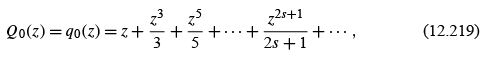we have A0 = 0, B= 1. Similar results follow for n = 1. We obtain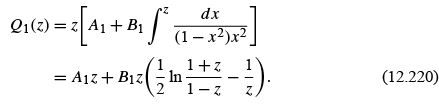Expanding in a power series and comparing with Q1 (z) =−p1 (z),wehave A1 = 0,B= 1. Therefore we may write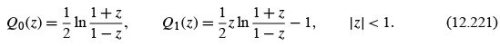Perhaps the best way of determining the higher-order Qn (z) is to use the recurrence relation (Eq. (12.17)), which may be veriﬁed for both x 2 < 1 and x 2 > 1 by substituting in the series forms. This recurrence relation technique yields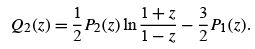(12.222)

Repeated application of the recurrence formula leads to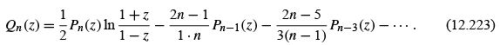From the form ln[(1 + z)/(1 − z)] it will be seen that for real z these expressions hold in the range −1 <x < 1. If we wish to have closed forms valid outside this range, we need only replace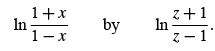When using the latter form, valid for large z, we take the line interval −1 ≤ x ≤ 1 as a cut line. Values of Qn (x ) on the cut line are customarily assigned by the relation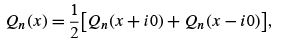(12.224)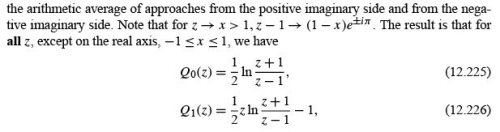and so on.
For convenient reference some special values of Qn (z) are given.

1. Qn (1) =∞, from the logarithmic term (Eq. (12.223)).

2. Qn (∞) = 0. This is best obtained from a representation of Qn (x ) as a series of negative powers of x ,

3. Qn (−z) = (−1)n+1 Qn (z). This follows from the series form. It may also be derived by using Q0 (z), Q1 (z) and the recurrence relation (Eq. (12.17)).

4. Qn (0) = 0, for n even, by (3).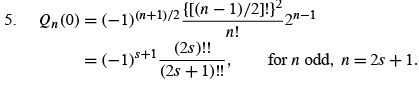This last result comes from the series form (Eq. (12.216)) with pn (0) = 1.

VECTOR SPHERICAL HARMONICS

Most of our attention in this chapter has been directed toward solving the equations of scalar ﬁelds, such as the electrostatic potential. This was done primarily because the scalar ﬁelds are easier to handle than vector ﬁelds. However, with scalar ﬁeld problems under ﬁrm control, more and more attention is being paid to vector ﬁeld problems.

Maxwell’s equations for the vacuum, where the external current and charge densities vanish, lead to the wave (or vector Helmholtz) equation for the vector potential A. In a partial wave expansion of A in spherical polar coordinates we want to use angular eigenfunctions that are vectors. To this end we write the coordinate unit vectors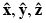in spherical notation (see Section 4.4),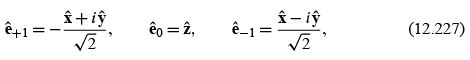so that eˆm form a spherical tensor of rank 1. If we couple the spherical harmonics with the eˆto total angular momentum J using the relevant Clebsch–Gordan coefﬁcients, we are led to the vector spherical harmonics: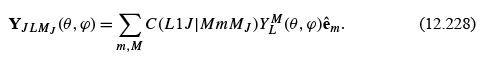It is obvious that they obey the orthogonality relations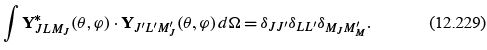Given J , the selection rules of angular momentum coupling tell us that L can only take on the values J + 1,J , and J − 1. If we look up the Clebsch–Gordan coefﬁcients and invert Eq. (12.228) we get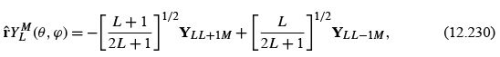displaying the vector character of the Y and the orbital angular momentum contents, L + 1 and L − 1,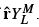Under the parity operations (coordinate inversion) the vector spherical harmonics transform as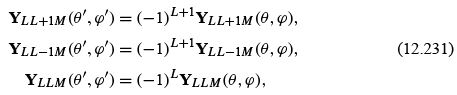where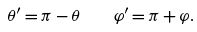(12.232)

The vector spherical harmonics are useful in a further development of the gradient (Eq. (2.46)), divergence (Eq. (2.47)) and curl (Eq. (2.49)) operators in spherical polar co-ordinates: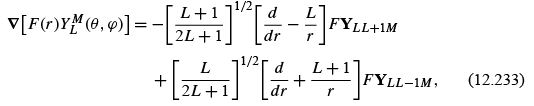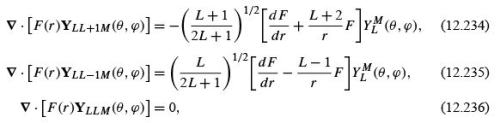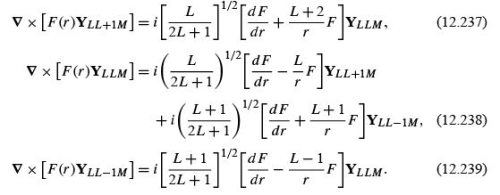If we substitute Eq. (12.230) into the radial component rˆ ∂/∂ r of the gradient operator, for example, we obtain both dF /dr terms in Eq. (12.233). For a complete derivation of Eqs. (12.233) to (12.239) . These relations play an important role in the partial wave expansion of classical and quantum electrodynamics.
The deﬁnitions of the vector spherical harmonics given here are dictated by convenience, primarily in quantum mechanical calculations, in which the angular momentum is a signiﬁcant parameter.

• Vector spherical harmonics are developed from coupling L units of orbital angular momentum and 1 unit of spin angular momentum. An extension, coupling L units of orbital angular momentum and 2 units of spin angular momentum to form tensor spherical harmonics, is presented by Mathews.
• The major application of tensor spherical harmonics is in the investigation of gravitational radiation.

Exercises

1.  Construct the l = 0,m = 0 and l = 1,m = 0 vector spherical harmonics.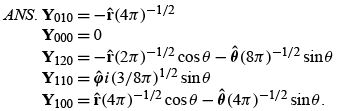159 docs

,

,

,

,

,

,

,

,

,

,

,

,

,

,

,

,

,

,

,

,

,

,

,

,

;